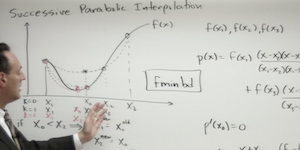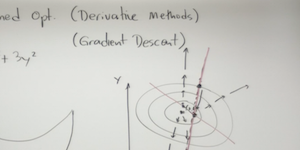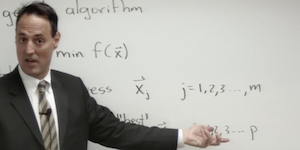# Beginning Scientific Computing

## Optimization

### Lecture 1### [ view ]

UNCONSTRAINED OPTIMIZATION (DERIVATIVE-FREE METHODS): We introduce some of the basic techniques of optimization that do not require derivative information from the function being optimized, including golden section search and successive parabolic interpolation.

MATLAB COMMANDS

`FMINBND`

### Lecture 2### [ view ]

UNCONSTRAINED OPTIMIZATION (DERIVATIVE METHODS): Derivative-based methods are some of the work-horse algorithms of modern optimization, including gradient descent.

MATLAB COMMANDS

`FMINSEARCH`

### Lecture 3### [ view ]

LINEAR PROGRAMMING AND GENETIC ALGORITHMS: We consider a number of more advanced optimization algorithms that include the genetic algorithm and linear programming for constrained optimization.

MATLAB COMMANDS

`LINPROG GA`

MATLAB CODE

© 2015 kutz